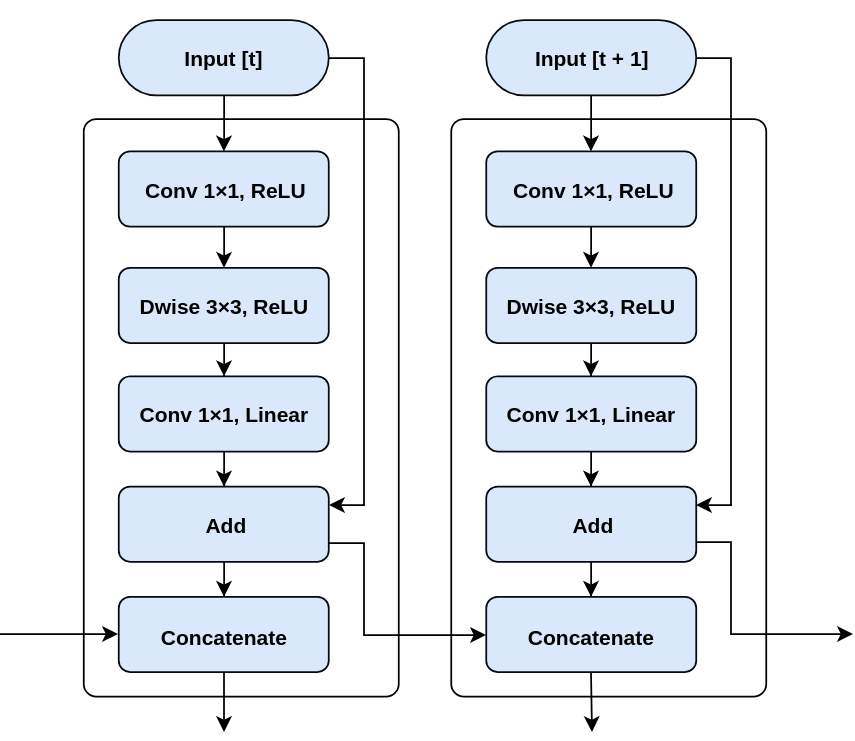/  Deep Learning Interview questions and answers   /  What do you mean by Mobile Net Model?## What do you mean by Mobile Net Model?

Mobile Net is an architecture which is more suitable for mobile and embedded based vision applications where there is lack of computation power. This architecture uses depth wise separable convolutions which significantly reduces the number of parameters when compared to the network with normal convolutions with the same depth in the networks. This results in light weight deep neural networks.

The normal convolution is replaced by depth wise convolution followed by pointwise convolution which is called as depth wise separable convolution.

In the normal convolution, if the input feature map is of Hi, Wi, Ci Hi, Wi, Ci dimension and we want Co feature maps with convolution kernel size KK then there are co convolution kernels each with dimension K, K, Ci K, K, Ci. This results in a feature map of Ho, Wo, Co Ho, Wo, Co dimension after convolution operation.

In the depth wise separable convolution, if the input feature map is of Hi, Wi, Ci Hi, Wi, Ci dimension and we want Co feature maps in the resulting feature map and the convolution kernel size is KK then there are Ci convolution kernels, one for each input channel, with dimension K,K,1K,K,1. This results in a feature map of Ho, Wo, Ci Ho, Wo, Ci after depth wise convolution. This is followed by pointwise convolution [1×1 convolution]. This convolution kernel is of dimension 1, 1, Ci1,1, Ci and there are Co different kernels which results in the feature map of Ho, Wo, Co Ho, Wo, Codimension.

This results in the reduction of number of parameters significantly and thereby reduces the total number of floating-point multiplications operations which is favorable in mobile and embedded vision applications with less compute power.

By using depth wise separable convolutions, there is some sacrifice of accuracy for low complexity deep neural network.